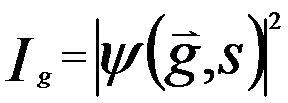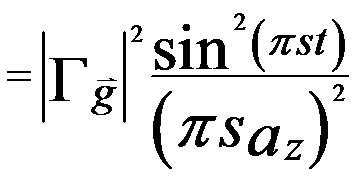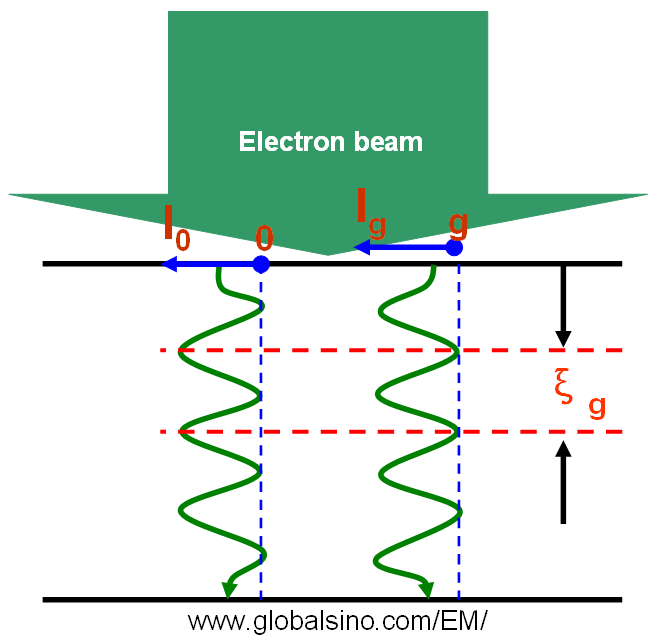Intensity of Diffracted Electron Beam in TEM
- Practical Electron Microscopy and Database -
- An Online Book -

https://www.globalsino.com/EM/

 This book (Practical Electron Microscopy and Database) is a reference for TEM and SEM students, operators, engineers, technicians, managers, and researchers. ================================================================================= Assuming the TEM specimen is a perfect crystal, in two beam condition the intensity (Ig) of diffracted electron beam can be given by,------------------------------ [4133a]------------------------- [4133b] where,           Γg -- Structure factor of the unit cell for diffraction g           s -- Deviation parameter           t -- Crystal thickness           az -- Lattice parameter along electron beam direction (z) According to dynamical theory, Equation 4133b can be re-written by,------------------------- [4133c]------------------------- [4133d]------------------------- [4133e] where,            seff -- Effective deviation parameter            ξg -- Extinction distance            V -- Volume of the unit cell            λ -- Electron wavelength Equation 4133c for dynamical theory is valid when the TEM sample is thick and s is about zero, but is not valid in kinematical theory. From Equation 4133e we can know that the extinction distance decreases with increase of scattering (increase of Γg). When s is equal to zero (exact Bragg condition) we have seff = 1/ξg, meaning the diffracted intensity (Ig) has a periodicity of ξg in TEM specimen depth indicated in Figure 4133 in two beam condition.Figure 4133. The diffracted intensity (Ig) showing a periodicity of ξg in TEM specimen depth in two beam condition. Ig is the intensity of transmitted beam. The exchange of intensity between the forward and diffracted beams in dynamical theory is called pendellösung. Pendellösung is not a kinematical effect because it does not allow such large change in intensity. Note that the intensities of the electron diffraction (ED) pattern from a crystalline specimen are independent of defocus and the spherical aberration of the electron-optical system. In EM (electron microscopy) crystallography, one method to quantum scattering is that the wave equation is mathematically converted to an integral form using the Green’s function. This integral equation is then expanded into Born series. As a first-order Born approximation, only the first term of the Born series is used. This is equivalent to assuming the incident electrons are scattered no more than once when penetrating through the specimen, called single, or kinematical, scattering. Using only the first term of the Born series also assumes that the directly transmitted electron wave can be reasonably approximated by the incident wave. In other words, the intensity of the diffracted waves is negligible compared to that of the direct wave. Note that the d-spacing and the intensities of the diffracted spots can be different for different crystals even though their space group are the same, for instance, as discussed in page3013 for space group Pnma (62).
=================================================================================

The book author (Yougui Liao) welcomes your comments, suggestions, and corrections, please click here for submission. If you let book author know once you have cited this book, the brief information of your publication will appear on the “Times Cited” page.